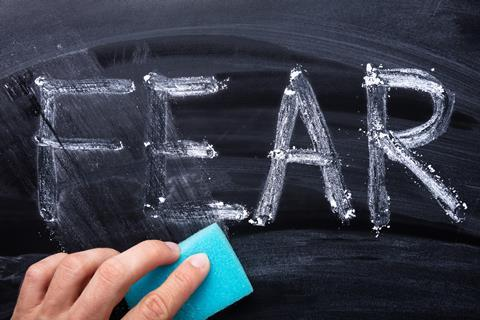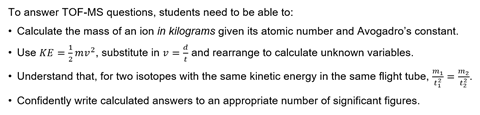Use these six steps to teach TOF-MS calculations and your students’ understanding and exam results will really take offThere’s no need to fret about time-of-flight

AQA introduced time-of-flight mass spectrometry (TOF-MS) calculations into its 2018 specification, accompanied by a student guide, example questions and answers and teaching notes. During a recent workshop with teachers, a number mentioned that there was a lack of available resources and the mathematical demand of the questions has left some teachers asking for support in teaching this content to students. Whether you teach to the AQA specification or not, TOF-MS calculations provide an ideal way to stretch high-attaining students, as well as potentially revealing any weaknesses in mathematical competence among your AS and A-level pupils.

### What’s the problem?

Examiners’ reports from the past couple of years identified that these questions ‘proved demanding for most students’ (AS-level paper, 1 June 2018) and ‘most students scored partial marks or no marks; common errors included failure to use the Avogadro constant, not converting mass to kg, and incorrect rearrangement of expressions’ (A-level paper, 1 June 2018). This article analyses the two types of question students can expect to encounter, highlighting common difficulties and suggesting approaches to improving mathematical fluency.

### The two types of question

In the first style of question, students are provided with information about an ion in the mass spectrometer and are expected to calculate a value for a variable about which no information has been given. For example, the question may provide information on an ion’s time of flight (t) and its kinetic energy (KE), and the student is expected to be able to calculate the length of the flight tube (d). The second style of question provides information about one isotope ion (eg 35Cl) and asks you to calculate the time of flight for a different isotope ion (eg 37Cl) with the same kinetic energy.

Both styles of question can be approached either through rearranging equations followed by minimal calculations or through a multistep approach that involves less algebra but requires more calculations. Both approaches have their strengths; the algebraic approach can be more demanding than the more accessible multistep approach, while the minimal calculations required reduce the potential for human error.### 6 steps to improve understanding and maths skills

#### 1. Chat to the physics and mathematics departments in your schools

Students are likely to think that these questions ‘feel’ a lot more like physics than chemistry, and those who are not studying maths or physics at AS/A-level may well find these types of questions more challenging. In addition, students who have relied on ‘triangles’ for managing equations at GCSE will likely feel out of depth. Chat with your physics and maths departments to understand when students encounter particular topics and how they develop their mathematical ability.

#### 2. Conduct an initial assessment of your students’ mathematical skill

A short maths quiz can be a good indicator of where students might be struggling, highlighting areas to target as well as providing a starting point for discussion with the maths and physics departments.

A suitable maths quiz (with answers) to assess your students’ skills as MS Word or pdf

#### 3. Develop familiarity with the equations before introducing numbers

The following questions may prove helpful in establishing student understanding. In addition, if students are confident in the kind of answers they expect to get, it can help them to spot mistakes in their calculations.

• What happens to the kinetic energy if I double the mass of the ball?
• What happens to the kinetic energy if I triple the velocity?
• What happens to the kinetic energy if I halve the velocity?
• What happens to the time of flight if I double the distance?

#### 4. Describe the equations in words

It’s easy for students to learn how to plug in the correct numbers, without ever needing to understand the equation or use it wisely. One way to develop familiarity is to ask students to describe the equation in words, using as much detail as possible.

For example, when discussing the kinetic energy of a 37Cl+ ion in the context of TOF-MS, a student might say: the kinetic energy of the 37Cl+ ion in Joules is equal to one half multiplied by the mass of the ion in kilograms, multiplied by the velocity of the ion in metres per second.

Talking through a worked example is a great way to model to students how you approach a question, particularly if you are explicit about the kinds of questions you might ask: what am I trying to find out? What do I already know? What equations will I be using? What units will I be working in?

It’s unfortunate that the equation for kinetic energy requires the mass to be expressed in kilograms, given that chemists work in grams for concentrations and molar calculations. Take a look at the kind of exercises you give to students. Can you ‘tweak’ them so that converting units becomes a more common feature of A- and AS-level problem solving?

A vague understanding of Avogadro’s constant can lead to students being able to calculate the mass of two moles of sodium, yet unable to calculate the mass of a single sodium atom or ion. To remedy this, consider introducing mass of atom/ion calculations alongside molar calculations

#### 6. Introduce the equations outside of a chemistry context

Have you considered teaching these calculations in the context of a bowling alley or a game of baseball? It’s possible that the chemistry context and the associated complications (numbers in standard form, unit conversion, significant figures) is actually masking something that students might find easier to understand in a different context.